## 4 回归

### 理解回归模型术语

• 响应(response)或因变量(dependent variable)：这些术语可以互用，表示基于其他一个或多个变量来预测的变量，通常使用y表示
• 解释变量(Explanatory variables)、自变量(independent variables)、特征(features)、属性(attributes)或回归系数(regressors)：这些术语可以互用，表示用于预测响应的变量，通常使用xx1 , x2表示
• 线性回归：该类型的回归会假设因变量会线性依赖自变量(即遵循直线方程)
• 非线性回归：该类型的回归会假设因变量会非线性依赖自变量(如多项式或指数)
• 多元回归(Multiple regression:)：具有超过一个自变量的回归
• 拟合(Fitting)或训练(training)：参数化模型的过程(如回归模型)，可以用该模型来预测一个特定的因变量
• 预测：使用参数模型预测因变量的过程(如回归模型)

### 线性回归

Mike Lee Williams (来自Fast Forward Labs的)说过:

#### 线性回归概述

$y = mx + b$

$sales = m * (number~of~users) + b$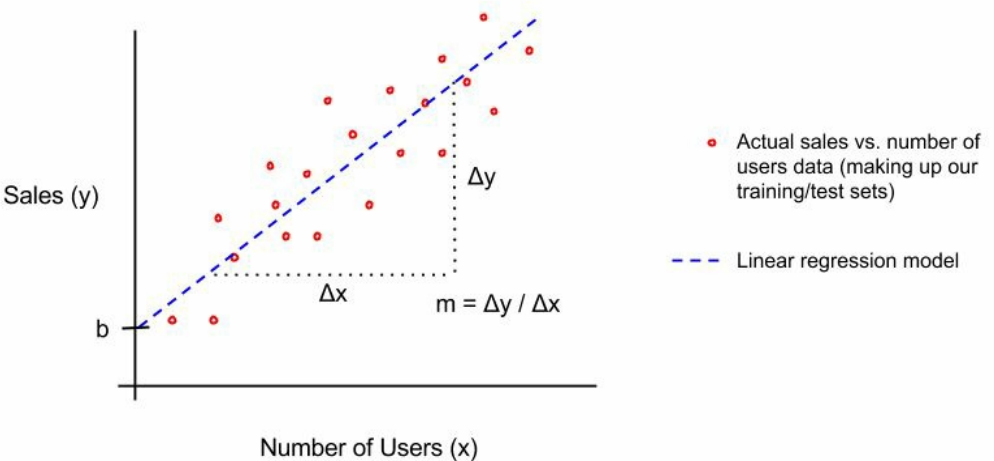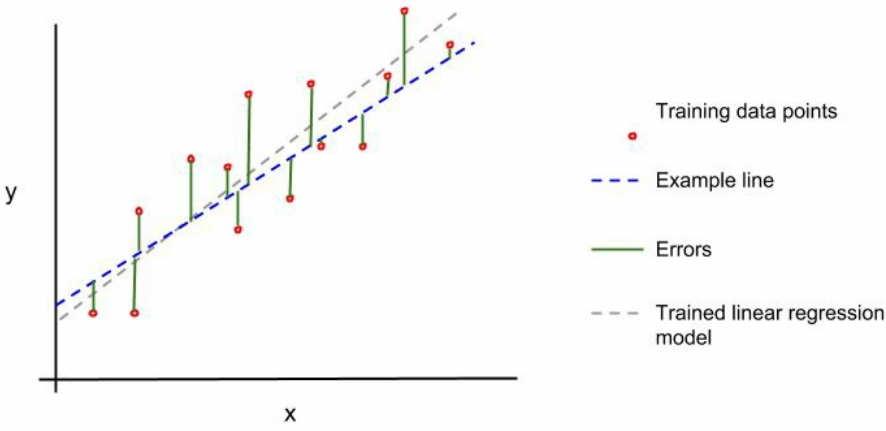$\frac{error^2_1+error^2_2+…+error^2_N}{N}$

#### 梯度下降法

// logisticRegression fits a logistic regression model
// for the given data.
func logisticRegression(features *mat64.Dense, labels []float64, numSteps int, learningRate f)
// Initialize random weights.
_, numWeights := features.Dims()
weights := make([]float64, numWeights)
s := rand.NewSource(time.Now().UnixNano())
r := rand.New(s)
for idx, _ := range weights {
weights[idx] = r.Float64()
}
// Iteratively optimize the weights.
for i := 0; i < numSteps; i++ {
// Initialize a variable to accumulate error for this iteration.
var sumError float64
// Make predictions for each label and accumulate error.
for idx, label := range labels {
// Get the features corresponding to this label.
featureRow := mat64.Row(nil, idx, features)
// Calculate the error for this iteration's weights.
pred := logistic(featureRow*weights + featureRow*weights)
predError := label - pred
sumError += math.Pow(predError, 2)
// Update the feature weights.
for j := 0; j < len(featureRow); j++ {
weights[j] += learningRate * predError * pred * (1 - pred) * featureRow[j]
}
}
}
return weights
}


// Iteratively optimize the weights注释下面的循环实现了通过SGD来优化逻辑回归参数。下面选择这部分循环来看下到底发生了什么。

// Calculate the error for this iteration's weights.
pred := logistic(featureRow*weights + featureRow*weights)
predError := label - pred
sumError += math.Pow(predError, 2)


$update=leaning~rate\times~gradient~of~the~parameters$

$parameter=parameters-update$

// Update the feature weights.
for j := 0; j < len(featureRow); j++ {
weights[j] += learningRate * predError * pred * (1 - pred) * featureRow[j]
}


gonum团队已经实现了梯度下降法：gonum.org/v1/gonum/optimize。文档地址：//pkg.go.dev/gonum.org/v1/gonum/optimize#GradientDescent

#### 线性回归的假设和缺点

• 线性关系：线性回归会假设因变量线性依赖自变量(线性方程)。如果这种关系不是线性的，则线性回归可能会表项不佳
• 正态性：假设变量遵循正太分布(看起来像钟形)。本章后面会讨论这种特性以及非正态分布变量下的取舍。
• 非多重共线性：多重共线性是一个特别的术语，它意味着自变量并不是真正独立的，它们会以某种形式相互依赖
• 没有自相关性：自相关性是另一个特别的术语，意味着变量依赖自身或自身的某个版本(如存在某些可预测的时序中)。
• 同方差性：这可能是这一组术语中最特别的一个，但它相对比较简单，且并不需要经常关注。线性回归假设回归线周围的数据的方差与自变量值的方差大致相同。

• 使用特定范围的自变量来训练线性回归模型，在预测该范围外的数据时应该格外小心，因为你的线性回归直线可能并不适用(如，某些极端数值下，因变量可能并不是线性的)。
• 可能为两个并无关联的变量建立了一个线性回归模型。需要确保变量之间有逻辑上的关联性。
• 可能会因为拟合某些特定类型数据中的异常或极端值而偏离回归线，如OLS。有一些方式可以让拟合回归不受异常值的影响，或针对异常值展示出不同的行为，如正交最小二乘法(orthogonal least squares)或岭回归(ridge regression)。

#### 线性回归的例子

$./myprogram [7x5] DataFrame column TV Radio Newspaper Sales 0: mean 147.042500 23.264000 30.554000 14.022500 1: stddev 85.854236 14.846809 21.778621 5.217457 2: min 0.700000 0.000000 0.300000 1.600000 3: 25% 73.400000 9.900000 12.600000 10.300000 4: 50% 149.700000 22.500000 25.600000 12.900000 5: 75% 218.500000 36.500000 45.100000 17.400000 6: max 296.400000 49.600000 114.000000 27.000000 <string> <float> <float> <float> <float>  上面以表格形式打印出所有的汇总数据，包括平均值、标准偏差、最小值、最大值、25%/75%百分位和中位数(或50%百分位)。 这些值为我们提供了良好的数值参考，后续会在训练线性回归模型时将看到这些数字。但缺乏直观上的理解，为此，我们需要为每列数值创建一个直方图： // Open the advertising dataset file. f, err := os.Open("Advertising.csv") if err != nil { log.Fatal(err) } defer f.Close() // Create a dataframe from the CSV file. advertDF := dataframe.ReadCSV(f) // Create a histogram for each of the columns in the dataset. for _, colName := range advertDF.Names() { // Create a plotter.Values value and fill it with the // values from the respective column of the dataframe. plotVals := make(plotter.Values, advertDF.Nrow()) for i, floatVal := range advertDF.Col(colName).Float() { plotVals[i] = floatVal } // Make a plot and set its title. p, err := plot.New() if err != nil { log.Fatal(err) } p.Title.Text = fmt.Sprintf("Histogram of a %s", colName) // Create a histogram of our values drawn // from the standard normal. h, err := plotter.NewHist(plotVals, 16) if err != nil { log.Fatal(err) } // Normalize the histogram. h.Normalize(1) // Add the histogram to the plot. p.Add(h) // Save the plot to a PNG file. if err := p.Save(4*vg.Inch, 4*vg.Inch, colName+"_hist.png"); err != nil { log.Fatal(err) } }  本程序会为每个直方图创建一个.png图像：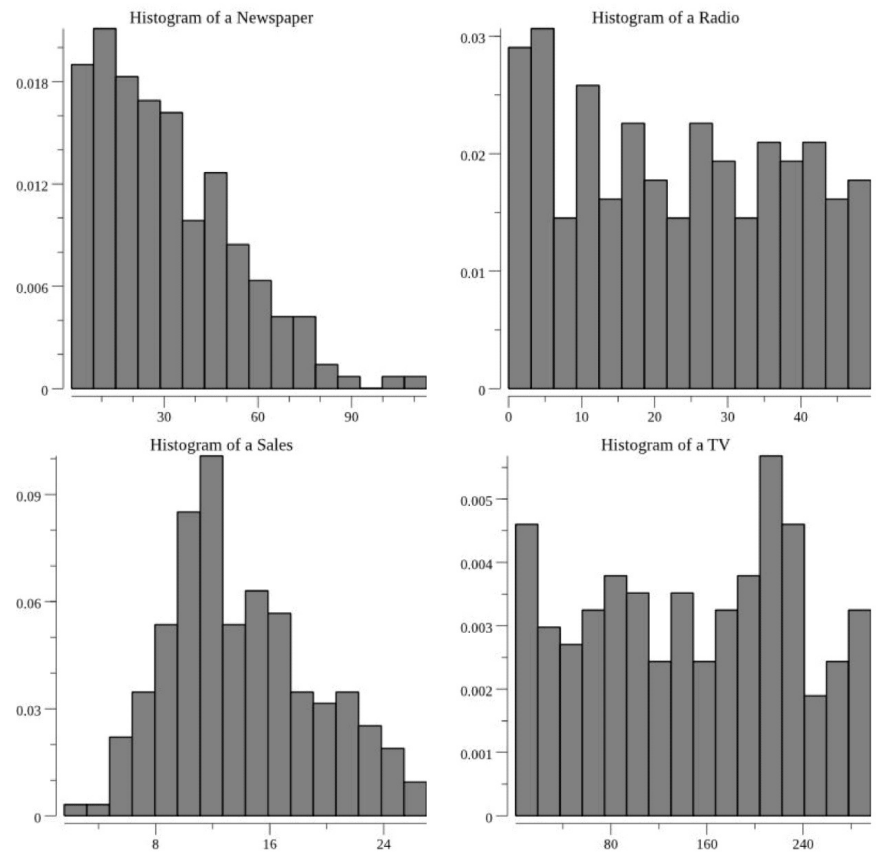观察上图以及计算出的汇总信息，下一步考虑是否符合线性回归的假设条件。可以看到并不是所有的变量都是正态分布的(钟形的)。可以看到销售额是钟形的，而其他则不是正态的。 我们可以使用分位图(quantile-quantile (q-q) p)统计工具来确定分布与正态分布的接近程度，甚至通过统计测试来确定变量是否服从正态分布的概率。但大多数情况下，通过图表就可以得出一个大致的结论。 下一步要做出决策，但至少有一部分数据在技术上并不会拟合到我们的线性回归模型中，可以选择如下一种方式进行处理： • 尝试转换变量，使其遵循正态分布，并在线性回归模型中使用这些转换的变量。这种方式的好处是可以在模型的假设中进行操作，缺点是可能会让模型难以理解，降低可解释性 • 使用不同的数据来解决问题 • 在线性回归假设中忽略该问题，并尝试创建该模型 可能还有其他解决问题的方式，但我的建议是首先尝试第三种选项。由于可以快速地训练线性回归模型，因此该选项并不会带来多少坏处。如果最后得出满意的模型，那么就可以避免引入更多的复杂性。如果得到的模型不尽如人意，那么此时再诉诸于其他选项。 ##### 选择自变量 现在对我们的数据有了一些直觉上的了解，并且已经了解到数据是如何拟合线性回归模型的假设的。那么现在应该选择哪个变量作为我们的自变量来预测因变量？ 最简单的方法是通过直观地探索因变量和选择的所有自变量之间的相关性，特别是可以通过绘制因变量与其他每个变量的散点图(使用pkg.go.dev/gonum.org/v1/plot)来做决定： // Open the advertising dataset file. f, err := os.Open("Advertising.csv") if err != nil { log.Fatal(err) } defer f.Close() // Create a dataframe from the CSV file. advertDF := dataframe.ReadCSV(f) // Extract the target column. yVals := advertDF.Col("Sales").Float() // Create a scatter plot for each of the features in the dataset. for _, colName := range advertDF.Names() { // pts will hold the values for plotting pts := make(plotter.XYs, advertDF.Nrow()) // Fill pts with data. for i, floatVal := range advertDF.Col(colName).Float() { pts[i].X = floatVal pts[i].Y = yVals[i] } // Create the plot. p, err := plot.New() if err != nil { log.Fatal(err) } p.X.Label.Text = colName p.Y.Label.Text = "y" p.Add(plotter.NewGrid()) s, err := plotter.NewScatter(pts) if err != nil { log.Fatal(err) } s.GlyphStyle.Radius = vg.Points(3) // Save the plot to a PNG file. p.Add(s) if err := p.Save(4*vg.Inch, 4*vg.Inch, colName+"_scatter.png"); err != nil { log.Fatal(err) } }  如此可以创建如下散点图：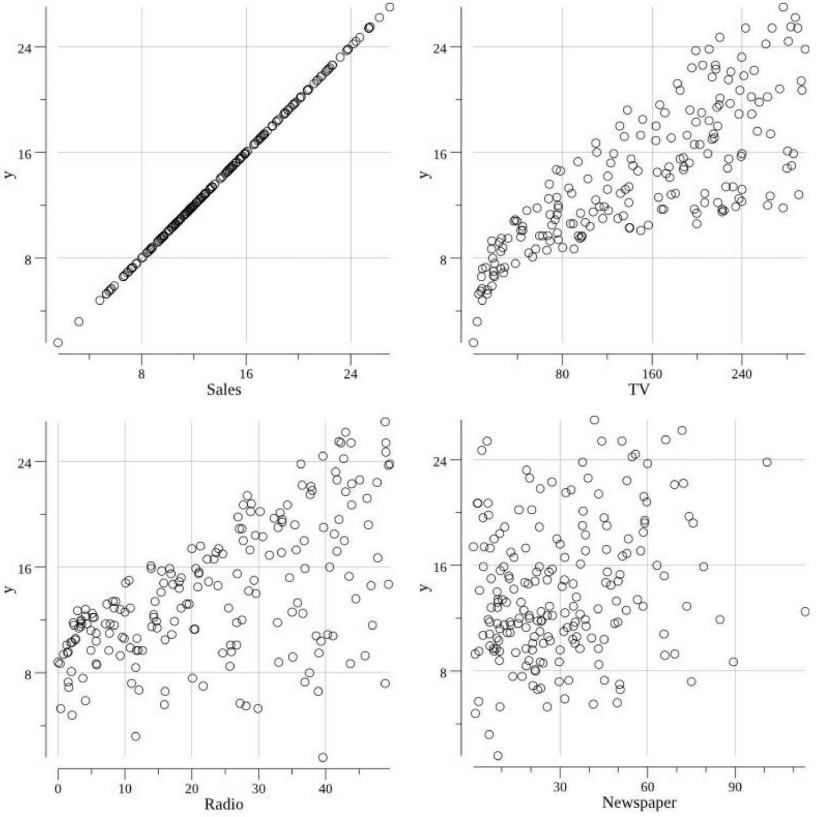通过这些散点图，我们需要推断出哪些属性 (TV, Radio, 和/或 Newspaper)与我们的因变量(Sales)具有线性关系。是否可以在这些散点图上画一条线，以符合销售趋势和各自的属性？这种方法并不总是行得通，且对于一个特定的问题，并不一定可以将其关联到所有的属性。 上述场景中，RadioTVSales呈线性关系，Newspaper可能与Sales有一定的关系，但相关性并不明显。与TV的相关性是最明显的，因此先选择TV作为线性回归模型的自变量，线性回归公式如下： $Sales = m~TV+b$ 这里要注意的另一件事是，变量TV可能不是严格等方差的(在线性回归的假设中讨论过)。这一点需要注意(可能值得在项目中归档的)，下面将继续探究是否可以创建具有预测能力的线性回归模型。当模型表现不佳时，需要重新审视这种假设。 ##### 创建训练和测试集 为了避免过度拟合并保证模型的推广，我们需要将数据集划分为训练集和测试集即评估和验证(Evaluation and Validation)。这里我们不会聚焦某个测试集，因为只需要进行一次模型训练即可，而不会在训练和测试之间来回迭代。但如果需要多个因变量进行验证和/或需要迭代调整模型参数时，你可能希望创建一个保留集，保存到模型开发过程结束后进行验证。 我们将使用github.com/go-gota/gota/blob/master/dataframe创建训练和测试数据集，并将它们保存到各自的.csv文件中。该场景中，我们使用80/20的比例来划分训练和测试数据： // Open the advertising dataset file. f, err := os.Open("Advertising.csv") if err != nil { log.Fatal(err) } defer f.Close() // Create a dataframe from the CSV file. // The types of the columns will be inferred. advertDF := dataframe.ReadCSV(f) // Calculate the number of elements in each set. trainingNum := (4 * advertDF.Nrow()) / 5 testNum := advertDF.Nrow() / 5 if trainingNum+testNum < advertDF.Nrow() { trainingNum++ } // Create the subset indices. trainingIdx := make([]int, trainingNum) testIdx := make([]int, testNum) // Enumerate the training indices. for i := 0; i < trainingNum; i++ { trainingIdx[i] = i } // Enumerate the test indices. for i := 0; i < testNum; i++ { testIdx[i] = trainingNum + i } // Create the subset dataframes. trainingDF := advertDF.Subset(trainingIdx) testDF := advertDF.Subset(testIdx) // Create a map that will be used in writing the data // to files. setMap := map[int]dataframe.DataFrame{ 0: trainingDF, 1: testDF, } // Create the respective files. for idx, setName := range []string{"training.csv", "test.csv"} { // Save the filtered dataset file. f, err := os.Create(setName) if err != nil { log.Fatal(err) } // Create a buffered writer. w := bufio.NewWriter(f) // Write the dataframe out as a CSV. if err := setMap[idx].WriteCSV(w); err != nil { log.Fatal(err) } }  上述代码会输出如下训练和测试集： $ wc -l *.csv
41  test.csv
161 training.csv
403 total


##### 训练模型

// Open the training dataset file.
f, err := os.Open("training.csv")
if err != nil {
log.Fatal(err)
}
defer f.Close()
// Read in all of the CSV records
if err != nil {
log.Fatal(err)
}
// In this case we are going to try and model our Sales (y)
// by the TV feature plus an intercept. As such, let's create
// the struct needed to train a model using github.com/sajari/regression.
var r regression.Regression
r.SetObserved("Sales")
r.SetVar(0, "TV")
// Loop of records in the CSV, adding the training data to the regression value.
for i, record := range trainingData {
if i == 0 {
continue
}
// Parse the Sales regression measure, or "y".
yVal, err := strconv.ParseFloat(record, 64)
if err != nil {
log.Fatal(err)
}
// Parse the TV value.
tvVal, err := strconv.ParseFloat(record, 64)
if err != nil {
log.Fatal(err)
}
// Add these points to the regression value.
r.Train(regression.DataPoint(yVal, []float64{tvVal}))
}
// Train/fit the regression model.
r.Run()
// Output the trained model parameters.
fmt.Printf("\nRegression Formula:\n%v\n\n", r.Formula)


$go build$ ./myprogram

Regression Formula:
Predicted = 7.07 + TV*0.05


##### 评估训练模型

// Open the test dataset file.
f, err = os.Open("test.csv")
if err != nil {
log.Fatal(err)
}
defer f.Close()
// Read in all of the CSV records
if err != nil {
log.Fatal(err)
}
// Loop over the test data predicting y and evaluating the prediction
// with the mean absolute error.
var mAE float64
for i, record := range testData {
if i == 0 {
continue
}
// Parse the observed Sales, or "y".
yObserved, err := strconv.ParseFloat(record, 64)
if err != nil {
log.Fatal(err)
}
// Parse the TV value.
tvVal, err := strconv.ParseFloat(record, 64)
if err != nil {
log.Fatal(err)
}
// Predict y with our trained model.
yPredicted, err := r.Predict([]float64{tvVal})
// Add the to the mean absolute error.
mAE += math.Abs(yObserved-yPredicted) / float64(len(testData))
}
// Output the MAE to standard out.
fmt.Printf("MAE = %0.2f\n\n", mAE)


$go build$ ./myprogram

Regression Formula:
Predicted = 7.07 + TV*0.05

MAE = 3.01


// predict uses our trained regression model to made a prediction.
func predict(tv float64) float64 {
return 7.07 + tv*0.05
}


// Open the advertising dataset file.
if err != nil {
log.Fatal(err)
}
defer f.Close()
// Create a dataframe from the CSV file.
// Extract the target column.
// pts will hold the values for plotting.
// ptsPred will hold the predicted values for plotting.
// Fill pts with data.
for i, floatVal := range advertDF.Col("TV").Float() {
pts[i].X = floatVal
pts[i].Y = yVals[i]
ptsPred[i].X = floatVal
ptsPred[i].Y = predict(floatVal)
}
// Create the plot.
p, err := plot.New()
if err != nil {
log.Fatal(err)
}
p.X.Label.Text = "TV"
p.Y.Label.Text = "Sales"
// Add the scatter plot points for the observations.
s, err := plotter.NewScatter(pts)
if err != nil {
log.Fatal(err)
}
// Add the line plot points for the predictions.
l, err := plotter.NewLine(ptsPred)
if err != nil {
log.Fatal(err)
}
l.LineStyle.Width = vg.Points(1)
l.LineStyle.Dashes = []vg.Length{vg.Points(5), vg.Points(5)}
// Save the plot to a PNG file.
if err := p.Save(4*vg.Inch, 4*vg.Inch, "regression_line.png"); err != nil {
log.Fatal(err)
}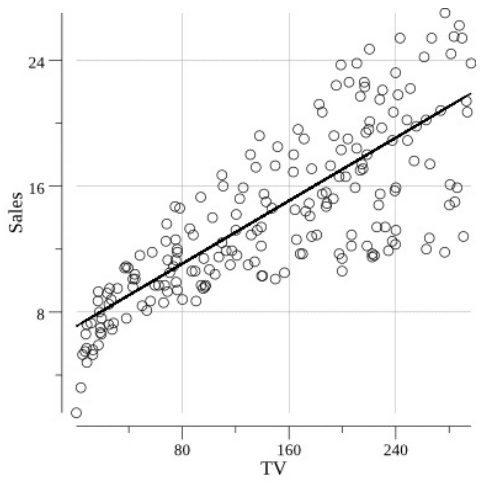#### 多元线性回归

$y=m_1x_1+m_1x_2+…+m_Nx_N+b$

• 过拟合：通过为模型添加越来越多的自变量，会增加模型的复杂度，并存在过拟合的风险。可以使用之前推荐的技术：正则化(regularization)来解决这种问题。 正则化在模型中创建一个惩罚项，该惩罚项是一个与模型复杂性有关的函数，有助于控制这种影响。
• 相对比例(Relative Scale)：在某些场景下，如果其中某个自变量的比例与另一个自变量的比例相差几个数量级，那么较大的变量可能会抵消较小变量带来的影响，因此可能需要考虑规范化变量。

$Sales=m_1TV+m_2Radio+b$

// Open the training dataset file.
f, err := os.Open("training.csv")
if err != nil {
log.Fatal(err)
}
defer f.Close()
// Read in all of the CSV records
if err != nil {
log.Fatal(err)
}
// In this case we are going to try and model our Sales
// by the TV and Radio features plus an intercept.
var r regression.Regression
r.SetObserved("Sales")
r.SetVar(0, "TV")
// Loop over the CSV records adding the training data.
for i, record := range trainingData {
if i == 0 {
continue
}
// Parse the Sales.
yVal, err := strconv.ParseFloat(record, 64)
if err != nil {
log.Fatal(err)
}
// Parse the TV value.
tvVal, err := strconv.ParseFloat(record, 64)
if err != nil {
log.Fatal(err)
}
if err != nil {
log.Fatal(err)
}
// Add these points to the regression value.
}
// Train/fit the regression model.
r.Run()
// Output the trained model parameters.
fmt.Printf("\nRegression Formula:\n%v\n\n", r.Formula)


$go build$ ./myprogram

Regression Formula:
Predicted = 2.93 + TV*0.05 + Radio*0.18


// Open the test dataset file.
f, err = os.Open("test.csv")
if err != nil {
log.Fatal(err)
}
defer f.Close()
// Read in all of the CSV records
if err != nil {
log.Fatal(err)
}
// Loop over the test data predicting y and evaluating the prediction
// with the mean absolute error.
var mAE float64
for i, record := range testData {
if i == 0 {
continue
}
// Parse the Sales.
yObserved, err := strconv.ParseFloat(record, 64)
if err != nil {
log.Fatal(err)
}
// Parse the TV value.
tvVal, err := strconv.ParseFloat(record, 64)
if err != nil {
log.Fatal(err)
}
if err != nil {
log.Fatal(err)
}
// Predict y with our trained model.
// Add the to the mean absolute error.
mAE += math.Abs(yObserved-yPredicted) / float64(len(testData))
}
// Output the MAE to standard out.
fmt.Printf("MAE = %0.2f\n\n", mAE)


$go build$ ./myprogram

Regression Formula:
Predicted = 2.93 + TV*0.05 + Radio*0.18
MAE = 1.26


#### 非线性以及其他类型的回归

$Sales=m_1TV+m_2TV^2+m_3TV^3+…+b$

github.com/berkmancenter/ridge中实现了Go语言的岭回归。与 github.com/sajari/regression不同，我们的自变量和因变量数据是通过gonum矩阵输入github.com/berkmancenter/ridge的。为了说明该方法，我们首先构造一个包含广告支出 (TV, Radio, 和Newspaper)的矩阵，以及包含Sales数据的矩阵。注意在github.com/berkmancenter/ridge中，如果想在模型中有一个截距，则需要为截距的输入自变量矩阵显式地添加一列，该列中的每个值仅为1.0

// Open the training dataset file.
f, err := os.Open("training.csv")
if err != nil {
log.Fatal(err)
}
defer f.Close()
// Read in all of the CSV records
if err != nil {
log.Fatal(err)
}
// featureData will hold all the float values that will eventually be
// used to form our matrix of features.
featureData := make([]float64, 4*len(rawCSVData))
yData := make([]float64, len(rawCSVData))
// featureIndex and yIndex will track the current index of the matrix values.
var featureIndex int
var yIndex int
// Sequentially move the rows into a slice of floats.
for idx, record := range rawCSVData {
if idx == 0 {
continue
}
// Loop over the float columns.
for i, val := range record {
// Convert the value to a float.
valParsed, err := strconv.ParseFloat(val, 64)
if err != nil {
log.Fatal(err)
}
if i < 3 {
// Add an intercept to the model.
if i == 0 {
featureData[featureIndex] = 1
featureIndex++
}
// Add the float value to the slice of feature floats.
featureData[featureIndex] = valParsed
featureIndex++
}
if i == 3 {
// Add the float value to the slice of y floats.
yData[yIndex] = valParsed
yIndex++
}
}
}
// Form the matrices that will be input to our regression.
features := mat64.NewDense(len(rawCSVData), 4, featureData)
y := mat64.NewVector(len(rawCSVData), yData)


// Create a new RidgeRegression value, where 1.0 is the
// penalty value.
r := ridge.New(features, y, 1.0)
// Train our regression model.
r.Regress()
// Print our regression formula.
c1 := r.Coefficients.At(0, 0)
c2 := r.Coefficients.At(1, 0)
c3 := r.Coefficients.At(2, 0)
c4 := r.Coefficients.At(3, 0)
fmt.Printf("\nRegression formula:\n")
fmt.Printf("y = %0.3f + %0.3f TV + %0.3f Radio + %0.3f Newspaper\n\n", c1, c2, c3, c4)


$go build$ ./myprogram
Regression formula:
y = 3.038 + 0.047 TV + 0.177 Radio + 0.001 Newspaper


// predict uses our trained regression model to made a prediction based on a
// TV, Radio, and Newspaper value.
func predict(tv, radio, newspaper float64) float64 {
return 3.038 + tv*0.047 + 0.177*radio + 0.001*newspaper
}


// Open the test dataset file.
f, err := os.Open("test.csv")
if err != nil {
log.Fatal(err)
}
defer f.Close()
// Read in all of the CSV records
if err != nil {
log.Fatal(err)
}
// Loop over the holdout data predicting y and evaluating the prediction
// with the mean absolute error.
var mAE float64
for i, record := range testData {
if i == 0 {
continue
}
// Parse the Sales.
yObserved, err := strconv.ParseFloat(record, 64)
if err != nil {
log.Fatal(err)
}
// Parse the TV value.
tvVal, err := strconv.ParseFloat(record, 64)
if err != nil {
log.Fatal(err)
}
if err != nil {
log.Fatal(err)
}
// Parse the Newspaper value.
newspaperVal, err := strconv.ParseFloat(record, 64)
if err != nil {
log.Fatal(err)
}
// Predict y with our trained model.
// Add the to the mean absolute error.
mAE += math.Abs(yObserved-yPredicted) / float64(len(testData))
}
// Output the MAE to standard out.
fmt.Printf("\nMAE = %0.2f\n\n", mAE)


$go build$ ./myprogram

MAE = 1.26